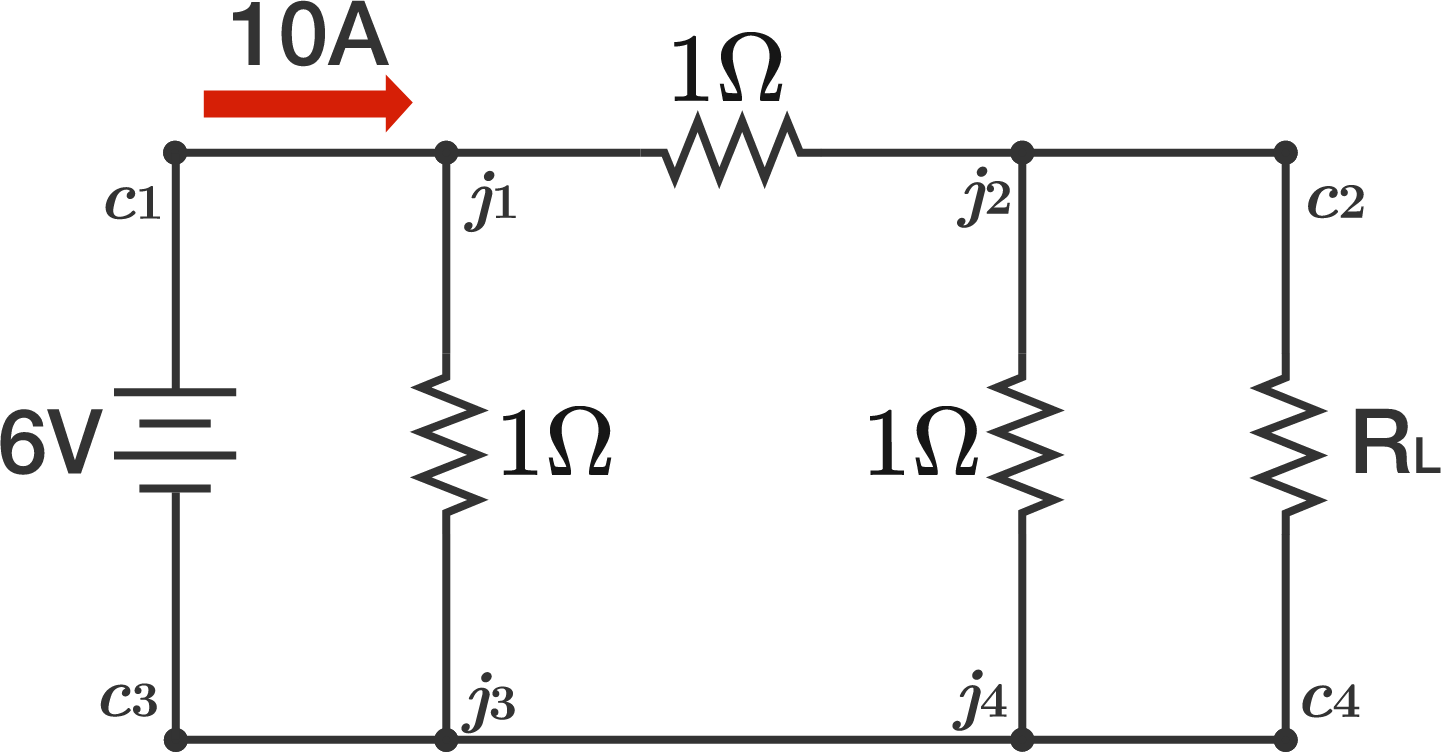# Pi Circuit AnalysisA $\pi$-circuit consisting of $\SI{1}{\ohm}$ resistors is connected to an ideal $\SI{6}{\volt}$ DC source at one pair of terminals, and to a load resistance $R_L$ at the other pair of terminals.

If $\SI{10}{\ampere}$ of current is flowing from the source, what is the value of $R_L$ in $\Omega ?$

×

Problem Loading...

Note Loading...

Set Loading...